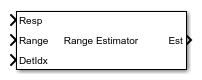# Range Estimator

Range estimation

• Library:
• Phased Array System Toolbox / Detection

•## Description

The Range Estimator block estimates the range of target detections obtained from the radar response data.

## Ports

### Input

expand all

Range-processed response data cube, specified as a complex-valued P-by-1 column vector, a complex-valued M-by-P matrix, or a complex-valued M-by-N-by-P array. M represents the number of range samples, N is the number of sensor elements or beams, and P is the number of Doppler bins.

The size of the first dimension of the input matrix can vary to simulate a changing signal length. A size change can occur, for example, in the case of a pulse waveform with variable pulse repetition frequency.

Data Types: `double`
Complex Number Support: Yes

Range grid values along the range dimension of the data cube input, Resp, specified as a real-valued M-by-1 column vector. Range values must be monotonically increasing and equally spaced. Units are in meters.

Example: `[-0.3,-0.2,-0.1,0,0.1,0.2,0.3]`

Data Types: `double`

Detection indices, specified as a real-valued Nd-by-Q matrix. Q is the number of detections and Nd is the number of dimensions in the response data cube, Resp. Each column of DetIdx contains the indices of a detection in the response data cube.

Noise power at detection locations, specified as a positive scalar or real-valued 1-by-Q row vector positive values. Q is the number of detections specified in the DetIdx input port.

#### Dependencies

To enable this port, select the Output variance for parameter estimates parameter, and then set Source of noise power parameter to `Input port`.

Cluster IDs, specified as a real-valued 1-by-Q row vector, where Q is the number of detections specified in the DetIdx input port. Each element of Clusters corresponds to an element of DetIdx.

#### Dependencies

To enable this input port, select the Enable cluster ID input checkbox.

### Output

expand all

Range estimates, specified as a real-valued K-by-1 column vector.

• When Enable cluster ID input is not selected, each range estimate corresponds to one of the columns of the DetIdx input port. Then K equals the column dimension, Q, of DetIdx.

• When Enable cluster ID input is selected, each range estimate corresponds to one of the cluster IDs in the Clusters input port. Then K equals the number of unique cluster IDs.

Range estimation variance, returned as a positive, real-valued K-by-1 column vector, where K is the dimension of Est. Each element of Var corresponds to an element of Est. The estimator variance is computed using the Ziv-Zakai bound.

#### Dependencies

To enable this output port, select the Output variance for parameter estimates parameter.

## Parameters

expand all

The maximum number of estimates to report, specified as a positive integer. When the number of requested estimates is greater than the number elements in DetIdx, the remainder is filled with `NaN`.

Data Types: `double`

Enable the Cluster input port to pass in cluster association information.

Data Types: `Boolean`

Enables the output of the parameter estimate variances via the Var port.

Data Types: `Boolean`

Root-mean-square range resolution of the detection, specified as a positive scalar. This parameter must have the same units as the Range input port.

#### Dependencies

To enable this parameter, select the ```Output variance for parameter estimates``` parameter.

Data Types: `double`

Source of the noise power, specified as `Property` or ```Input port```. If you set this parameter to `Property`, use the Noise power parameter to set the noise power at the detection locations. When set the parameter to ```Input port```, specify noise power via the `NoisePower` input port.

Noise power for detections, specified as a positive scalar. The same noise power value is applied to all detections. Noise power is in linear units.

#### Dependencies

To enable this parameter, select the Output variance for parameter estimates checkbox and set the Source of noise power parameter to `Property`.

Data Types: `double`

Block simulation, specified as `Interpreted Execution` or ```Code Generation```. If you want your block to use the MATLAB® interpreter, choose `Interpreted Execution`. If you want your block to run as compiled code, choose `Code Generation`. Compiled code requires time to compile but usually runs faster.

Interpreted execution is useful when you are developing and tuning a model. The block runs the underlying System object™ in MATLAB. You can change and execute your model quickly. When you are satisfied with your results, you can then run the block using ```Code Generation```. Long simulations run faster with generated code than in interpreted execution. You can run repeated executions without recompiling, but if you change any block parameters, then the block automatically recompiles before execution.

This table shows how the Simulate using parameter affects the overall simulation behavior.

When the Simulink® model is in `Accelerator` mode, the block mode specified using Simulate using overrides the simulation mode.

Acceleration Modes

 Block Simulation Simulation Behavior `Normal` `Accelerator` `Rapid Accelerator` `Interpreted Execution` The block executes using the MATLAB interpreter. The block executes using the MATLAB interpreter. Creates a standalone executable from the model. `Code Generation` The block is compiled. All blocks in the model are compiled.